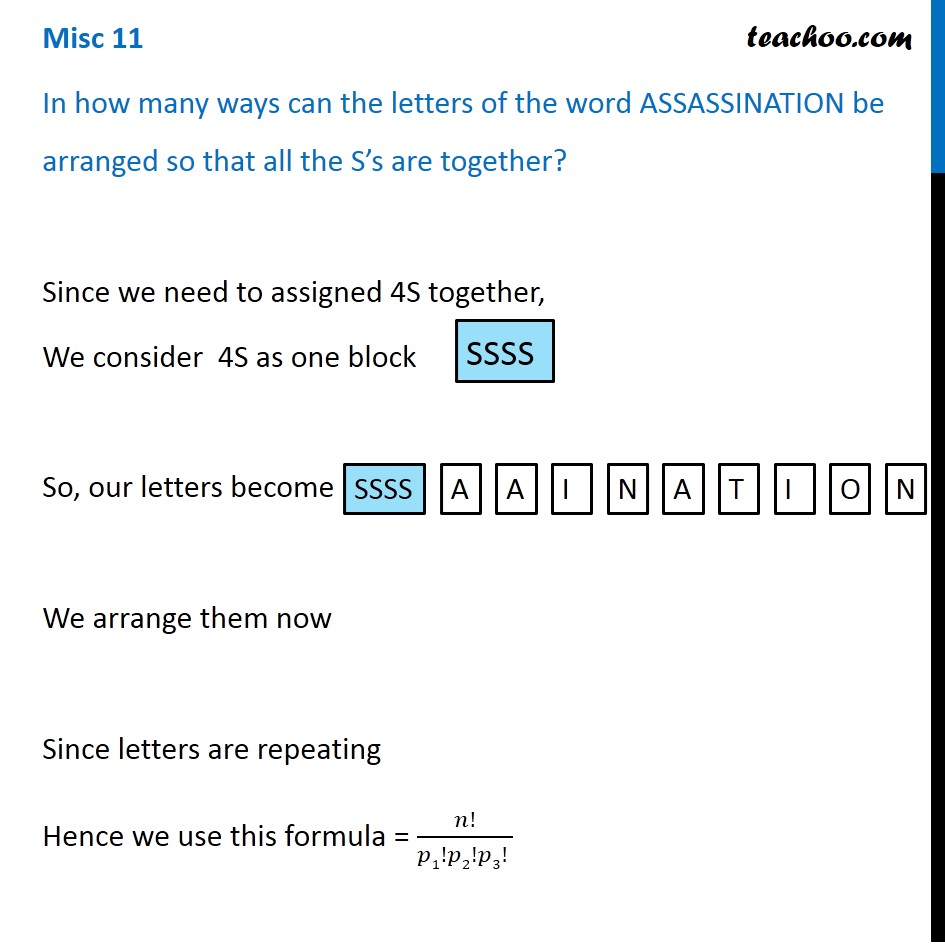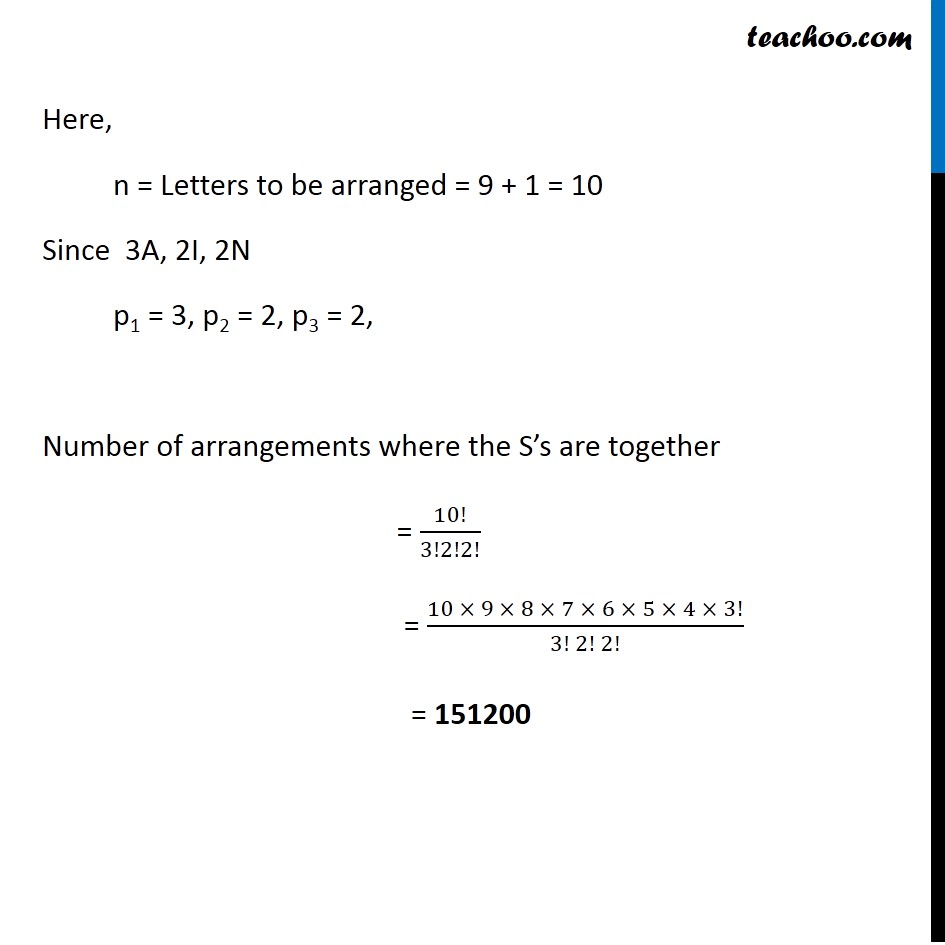Miscellaneous

Chapter 6 Class 11 Permutations and Combinations
Serial order wiseLearn in your speed, with individual attention - Teachoo Maths 1-on-1 Class

### Transcript

Misc 11 In how many ways can the letters of the word ASSASSINATION be arranged so that all the S’s are together? Since we need to assigned 4S together, We consider 4S as one block So, our letters become We arrange them now Since letters are repeating Hence we use this formula = 𝑛!/(𝑝1!𝑝2!𝑝3! ) Here, n = Letters to be arranged = 9 + 1 = 10 Since 3A, 2I, 2N p1 = 3, p2 = 2, p3 = 2, Number of arrangements where the S’s are together = 10!/3!2!2! = (10 × 9 × 8 × 7 × 6 × 5 × 4 × 3!)/(3! 2! 2!) = 151200